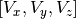# Modules for modeling of molecular structure¶

## Module for class ‘Structure’¶

There is a module, that realizes data structure - molecule and methods for its modification.

class kvazar.core.structure.Structure(struct_data, auto_calculate=True)[source]
• atom_names - Array of names of atoms, i.e. ‘Carbon’, ‘Hydrogen’ and etc.
• atom_types - Numbers, that are correspondind to atoms names
• n_atoms - Number of atoms in structure
• n_links - Number of maximum possible links for atom. The second dimension of links matrix
• coords - Array of coordinates of each atom in structure
• velocity - Array of atoms velocities in form• tension - Array of tensions for all atoms
• charges - Array of electric charge on all atoms
• links - Array of links of each atom with all others (one-sided links)
• num_links - Number of links for each atom
• full_links - Array of links for all atoms (two-sided bonds)
• num_full_links - Array of numbers of two-sided links for each atom
compare_topology_with(b)[source]

It only compares number of atoms, links and identity of atom types in two structures.

compare_with(b)[source]

If the structures are the same, it returns True.

copy()[source]

Returns new structure which is equal to the initial

It makes 2D array of reciprocal links between atoms.

It returns array of neighbours of all atoms, array of numbers of all atoms, full number of atoms

It finds sequences of atoms, that form dihedral angles

getsubstr(selected)[source]

It forms new structure from selected atoms. For example:

Some_structure.getsubstr([(0,20),(30,45)]) - it returns new object of class Structure, that is made from 0-20 and 30-45 atoms of initial structure.

tors_dfs(full_links, pos, used, cur_way, result)[source]

Depth-first search to find dihedral angles

update_neighbours()[source]

It updates neighbours matrix, matrix of number of neighbours

There are some overrided methods for structure, as __add__, __iadd__, __getslice__, __delitem__.

And here is a python code of example of usage of module:

struct_data = dict()
n_atoms = 2
struct_data['n_atoms'] = n_atoms
struct_data['atom_names'] = ['Carbon','Carbon']
struct_data['coords'] = numpy.zeros((n_atoms,3), numpy.float)
struct_data['coords'] = 1.42
struct_data['num_links'] = numpy.zeros(n_atoms, numpy.float)
A = structure.Structure(struct_data)
B = A.copy()
B.coords = B.coords = 1.42
C = A + B


You will get new structure with 4 atoms, where coordinates are concatenated, when velocities, links are still the same as in original A and B

A += B


You will get modified structure A (A = A + B)

D = A[1:2]


You will get new structure, which is a part of structure A and has 2 atoms (from 1 to 2 inclusive).

del A[[(0,0), (1,2)]]


It deletes atoms of structure: 0,1,2. It changes initial structure.

E = C.getsubstr([(1,2)])


You will get new structure, that made of atoms 1,2.

## Module of class for structure loading and saving¶

class kvazar.fileman.FileManager[source]

It’s a class for structure loading from files. Possible extensions of files for loading are ACD, ACC, PDB. Now there is only one possible extension for saving: ACD. Also class checks existance of direcory and makes the new one, if needed. Raise exception in the case of unknown extension.

There is an example of fileman.py usage in package:

from kvazar.fileman import FileManager
fm = FileManager()


It loads model of fullerene C28

fm.set_struct(struct1)
fm.save("path/to/c28_new.ACD")


It saves copy of model of fullerene in new file. Examples of input files can be found in kvazar’s directory: Path_to_kvazar + “/test/test_data/”

## Module for saving output file¶

class kvazar.record.Record(rec_file, rec_type='w')[source]

It’s a class for saving intermediate data of calculation and archive it in one output file.

It provides an interface to the molecular dynamic data. It allows:

• To load any state of calculated file. States are from 0 to the last of states
python
from kvazar.record import Record
rec = Record('path/to/file.nmd', 'a')
rec.update_state(1)

• To see parameters, that were calculated, for any state:
rec.params


There are two possible modifiers in initializing of object of class Record:

• ‘a’ - it will append new data to already existed
• ‘w’ - it will rewrite data